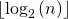# Practice Questions for Recursion | Set 2

• Difficulty Level : Easy
• Last Updated : 21 Aug, 2022

Explain the functionality of the following functions.

Question 1

## C++

 `/* Assume that n is greater than or equal to 1 */``int` `fun1(``int` `n)``{``    ``if` `(n == 1)``        ``return` `0;``    ``else``        ``return` `1 + fun1(n / 2);``}`

## Java

 `/* Assume that n is greater than or equal to 1 */``static` `int` `fun1(``int` `n)``{``    ``if` `(n == ``1``)``        ``return` `0``;``    ``else``        ``return` `1` `+ fun1(n / ``2``);``}`

## Python3

 `# Assume that n is greater than or equal to 1 */``def` `fun1(n):``    ``if``(n ``=``=` `1``):``        ``return` `0``    ``else``:``        ``return` `1` `+` `fun1(n``/``/``2``)`

## C#

 `/* Assume that n is greater than or equal to 1 */``static` `int` `fun1(``int` `n)``{``    ``if` `(n == 1)``        ``return` `0;``    ``else``        ``return` `1 + fun1(n / 2);``}`

## Javascript

 ``

Answer: The function calculates and returnsFor example, if n is between 8 and 15 then fun1() returns 3. If n is between 16 to 31 then fun1() returns 4.

Question 2

## C++

 `/* Assume that n is greater than or equal to 0 */``void` `fun2(``int` `n)``{``if``(n == 0)``    ``return``;` `fun2(n/2);``cout << n%2 << endl;``}`

## C

 `/* Assume that n is greater than or equal to 0 */``void` `fun2(``int` `n)``{``  ``if``(n == 0)``    ``return``;` `  ``fun2(n/2);``  ``printf``(``"%d"``, n%2);``} `

## Java

 `/* Assume that n is greater than or equal to 1 */``static` `void` `fun2(``int` `n)``{``if``(n == ``0``)``    ``return``;`` ` `fun2(n/``2``);``System.out.println(n%``2``);``}`

## Python3

 `# Assume that n is greater than or equal to 0 */``def` `fun2(n):``    ``if``(n ``=``=` `0``):``        ``return``    ` `    ``fun2(n ``/``/` `2``)``    ``print``(n ``%` `2``, end``=``"")`

## C#

 `void` `fun2(``int` `n)``{``if``(n == 0)``    ``return``;``  ` `fun2(n/2);``Console.Write(n%2);``}`

## Javascript

 ``

Auxiliary Space: O(1)

Answer: The function fun2() prints the binary equivalent of n. For example, if n is 21 then fun2() prints 10101.

Note: Above functions are just for practicing recursion, they are not the ideal implementation of the functionality they provide.

Please write comments if you find any of the answers/codes incorrect.

My Personal Notes arrow_drop_up